﻿ Predictive Modelling of Benign and Malignant Tumors Using Binary Logistic, Support Vector Machine and Extreme Gradient Boosting ModelsPublications are Open
Access in this journal
Article Versions
Export Article
• Normal Style
• MLA Style
• APA Style
• Chicago Style
Research Article
Open Access Peer-reviewed

### Predictive Modelling of Benign and Malignant Tumors Using Binary Logistic, Support Vector Machine and Extreme Gradient Boosting Models

Peter Gachoki , Moses Mburu, Moses Muraya
American Journal of Applied Mathematics and Statistics. 2019, 7(6), 196-204. DOI: 10.12691/ajams-7-6-2
Received October 14, 2019; Revised November 18, 2019; Accepted November 26, 2019

### Abstract

Breast cancer is the leading type of cancer among women worldwide, with about 2 million new cases and 627,000 deaths every year. The breast tumors can be malignant or benign. Medical screening can be used to detect the type of a diagnosed tumor. Alternatively, predictive modelling can also be used to predict whether a tumor is malignant or benign. However, the accuracy of the prediction algorithms is important since any incidence of false negatives may have dire consequence since a person cannot be put under medication, which can lead to death. Moreover, cases of false positives may subject an individual to unnecessary stress and medication. Therefore, this study sought to develop and validate a new predictive model based on binary logistic, support vector machine and extreme gradient boosting models in order to improve the prediction accuracy of the cancer tumors. This study used the Breast Cancer Wilcosin data set available on Kaggle. The dependent variable was whether a tumor is malignant or benign. The regressors were the tumor features such as radius, texture, area, perimeter, smoothness, compactness, concavity, concave points, symmetry and fractional dimension of the tumor. Data analysis was done using the R-statistical software and it involved, generation of descriptive statistics, data reduction, feature selection and model fitting. Before model fitting was done, the reduced data was split into the train set and the validation set. The results showed that the binary logistic, support vector machine and extreme gradient boosting models had predictive accuracies of 96.97%, 98.01% and 97.73%. This showed an improvement compared to already existing models. The results of this study showed that support vector machine and extreme gradient boosting have better prediction power for cancer tumors compared to binary logistic. This study recommends the use of support vector machine and extreme gradient boosting in cancer tumor prediction and also recommends further investigations for other algorithms that can improve prediction.

### 1. Introduction

Cases of breast cancer diagnosis and mortality have grown over the years across the world. This has been attributed to changes in lifestyles as well as hormonal changes 1. Mortalities from breast cancer have been attributed to late diagnosis as well as challenges in access to treatment. Studies have proposed increased campaign on self-examination that will facilitate early diagnosis which willing turn help in control and treatment of breast cancer 8. Breast cancer is caused by the buildup of extra cells of the on the breast causing a mass tissue that is usually referred to as a lump or a tumor. A tumor on the breast can either be malignant or begnin 3. A benign tumor is not cancerous while a malignant tumor is cancerous. Benign tumors are harmless and they do not cause an invasion to tissues next to them and neither do they spread to other body parts. When a benign tumor is removed, it does not grow back again. On the other hand malignant tumors are dangerous and they can affect the tissues next to them. These tumors can also spread to other body parts and even when they are removed, there is always a possibility that they will grow back 5.

Researchers have also tried to highlight some breast cancer risk factors. These include; gender, age, history, genes, radiations, ethnicity, overweight, breast feeding, alcoholism, nature of breasts, smoking, low levels of vitamin D, chemical exposure among others 2. Prediction of whether a tumor is benign or malignant can be an important step in breast cancer control. This is because if a tumor is predicted to be malignant, early medication can be sought and thus the cancer can be controlled before it gets to an advanced stage. However, the prediction accuracies and are very important since cases of false negatives may have dire consequences since somebody cannot be put under medication and this can lead to deaths. Cases of false positives can subject to unnecessary stress and medication. therefore, it is important to develop an algorithm that can predict if a tumor is begnin or malignant with the best accuracy as possible.

Several studies have come up with prediction algorithms for breast cancer, and have attained different prediction accuracies. For example a study that investigated whether breast cancer was caused by modifiable or non-modifiable factors using the Rep Tree, RBF Network and using simple logistic attained a classification accuracy rate of 74.5% 4. The non-modifiable factors considered were age, menustrial history, gender, age at menopause, age at menarche and number of first degree relatives who have suffered from breast cancer. The modifiable factors were BMI, number of children, alcoholism, diet, age at first birth and number of abortions. Prediction of whether a tumor was benign or malignant using Naïve Bayes, SVM-RBF kernel, decision tree, neural networks and regression tree produced the SVM-RBF kernel with an accuracy of 96.84% 5, when prediction of benign and malignant breast cancer was done using data mining techniques, Naïve Bayes attained the best prediction accuracy of 97.73%. Application of Decision Trees, Naive- Bayesian methods, Sequential Minimal Optimization to detect breast cancer tumors, Sequential Minimal Optimization showed high level performance compare with other classifiers 4.

It is clear that prediction accuracies of the prediction algorithms vary according to the algorithm used. Further, these accuracies could also vary depending on the pre analysis performed on the data. Such pre analysis includes the data imputations and the data dimension reductions if the variables are correlated. This study therefore sought to investigate if the binary logistic, support vector machine and extreme gradient boosting improved the prediction accuracies of the begnin and malignant tumors. The algorithms were developed after performing data imputation and data dimension reduction using the principal component analysis.

### 2. Methodology

This study used the breast cancer data available on Kaggle. The dependent variable in the data is whether a tumor is benign or malignant. The predictor variables are features of tumor that includes; radius, texture, area, perimeter, smoothness, compactness, concavity, concave points, symmetry and fractional dimension of the tumor. The analysis of the data involved generation of descriptive statistics, performing data reduction and feature selection using the principal component analysis and then fitting the binary logistic, support vector machine and extreme gradient boosting models. The data was split into two where 70% formed the training data set and 30% formed the testing set.

2.1. Principal Component Analysis

This is a procedure that makes use of the orthogonal transformation to convert correlated variables into linearly uncorrelated variables that are referred to as principal components 7. The first principal component has the largest possible variance and every subsequent component has the largest possible variance under the constraint that is orthogonal to the subsequent components. The end result is a vector of uncorrelated orthogonal basis set.

Mathematically, the transformation;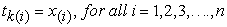is a set of vectors of coefficients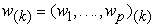that map every row vector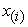of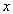to a new vector scores of principal components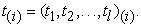This is done in such a way that the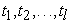of t considered over the data set inherit maximum possible variance from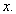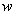is constrained to be a unit vector and the choice of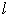is selected in such a way that it is less than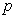so as to reduce dimensionality.

For maximum variance, the weight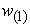must satisfy;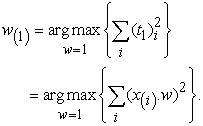Onceis obtained, the first principal component is given as;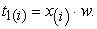The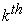principal component can be obtained through subtracting the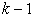principal components from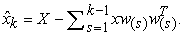The principal component analysis also helps in data dimension reduction while still retaining much of the variance in the data sets.

2.2. Logistic Regression

It is a generalized linear model that fits data that has a binary outcome. If the data has multiple classes, the logistic regression generalizes into a multinomial regression model (Sperandei, 2014). The logistic regression equation is;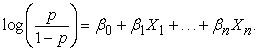Using the maximum likelihood estimation, the cost function can be derived as;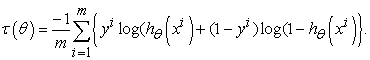The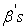are obtained by minimizing the cost function.

To solve the problem of over fitting when using a logistic regression, a regularized logistic regression is used. This is achieved by adding a regularization term to the cost function. The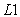regularization is achieved by adding a penalty that is equivalent to the sum of absolute values of the coefficients. That is;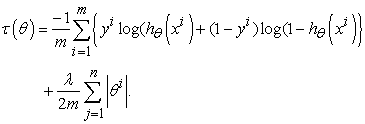To optimize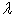cross-validation is used and thethat yields the best cross-validation accuracy is chosen.

2.3. Support Vector Machine

This is an algorithm under supervised machine learning that is used for classification and regression. However, most of the times, it is used for classification 9. To understand the working of support vector machine model, an example of a data is considered that has two classes that can be separated using a straight line which can also be referred to as the decision boundary or hyperplane (Figure 1).

• Figure 1. Data set with two classes that are separable using a straight line

The point under consideration is which is the best line that can separate the two classes since there are multiple lines that can do the separation. This consideration leads to the concept of maximum margin classification. This means that the support vector machine finds the hyperplane that yields the largest margin between the two classes.

Choosing the solid line as the hyperplane and margins as the dotted lines, the points (circled) that lie on the margin are referred to as support vectors (Figure 2).

• Figure 2. Diagrammatic representation of the support vectors

The support vectors are the ones that are used by the support vector machine to obtain a decision boundary. The others points are not used. Since for this case the space is two dimensional, the equation for the separating line is;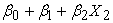When the equation evaluates to more than 0, then 1 is predicted. That is;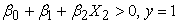When the equation evaluates to less than 0, then -1 class is predicted. That is;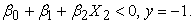This yields a maximization problem;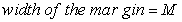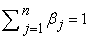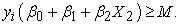In most cases, the classes are noisy. Considering a case where no matter the line chosen, some points will always be on the wrong side of the decision boundary, the maximum margin classification would not work (Figure 3).

• Figure 3. Diagrammatic representation of data with noisy classes

In such cases, support vector machine introduces a soft margin that allows some points to be on the wrong side. By introduction of the error term, some slack is allowed. An example of two case maximization yields;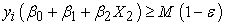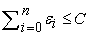Where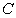is a tuning parameter that determines the width of the margin while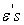are the slack variables that allow observations to fall on the wrong side of the margin (Figure 4).

• Figure 4. Support vector machine model classification for data with noisy classes.

If the decision boundary is non-linear, support vector machine introduces kernels.

2.4. XGBoost

XGBoost is an ensemble learner where the multiple machine learning algorithms are used at the same time for prediction 6. An example of an ensemble learner is the random forest that uses many decision trees for prediction. Ensemble learners are classified into Bagging and Boosting. The random forest is a bagging learner where decision trees are developed from the subsets of the training data set and the final prediction is a weighted sum of all the decision tree functions. In boosting learners, samples are selected sequentially. For instance, the first sample is selected and a decision tree is fitted. The model then picks the examples that were hard to learn and using them and a few others selected at random from the training data set, a second model is fitted. Prediction is then made using the first and the second models. The model is then evaluated and hard examples are picked together with other randomly selected examples from training set and another model is fitted. The process of boosting algorithms continues up to a number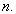In gradient boosting, the first model is fitted using the original training set. For example, a simple regression model,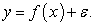If the error, say, it is too large, one might try to, say, add more features, use another algorithm, tune the algorithm, look for more training set etc. However, if the error is not white noise and it has a relationship with the output, then a second model can be fitted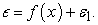The process continues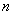– times and the final model will be;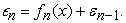The final step involves adding these models together with some weighting criteria;

Weights =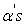which yields the final function that is used for prediction.

2.5. Model Comparison Criterions

Below is a presentation of the criterions that were used for model comparison;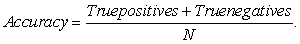Precision: this is a measure of the proportion of patients who were predicted to have a malignant tumor and actually had it.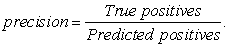Recall (sensitivity): this is a measure of the proportion of the patients that had malignant tumor and were detected by the predicting algorithm. This is referred to as the true positive rate.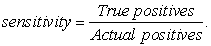Specificity is the true negative rate. This is the proportion the patients who had benign tumors and were detected by the predicting algorithm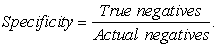### 3. Results and Discussion

3.1. Descriptive Statistics

The percentage of women with malignant tumors was 37.26% while the rest 62.74% had benign tumors. These percentages presented 212 out of 569 for malignant tumors and 357 out of 569 for begnin tumors (Figure 5).

• Figure 5. Percentages of women with benign and malignant tumors (B – Begnin tumor, M – Malignant tumor)
• Figure 6. Box plots for variables where there is a significant difference between the tumor types

From the boxplots, variables where there is a significant difference between the two groups of cancer tumors can be identified. When using a boxplot, if two distributions do not overlap or more than 75% of two boxplot do not overlap then a significant difference in the mean/median between the two groups is expected. Some of the variables where the distributions of two cancer tumors are significantly different are radius mean, texture mean among others. The visible differences between malignant tumors and benign tumors can be seen in means of all cells and worst means where worst means is the average of all the worst cells. The distributions of malignant tumors have higher scores than the benign tumors in these cases (Figure 6).

Some of the variables were highly correlated. Principal component analysis was used for data dimension reduction. Since variables were correlated it was evident that smaller set of features could be used in building of the models. The correlated variables were shown using a correlation matrix. An extract of the correlation matrix is presented in Table 1.

Using the elbow rule, the first 15 principle components were used. Using 15 principle components, almost 100% of the variance from the original data set was achieved. Since principal component analysis formed new characteristics, the variance explained plot was used to show the amount of variation of the original features captured by each principle component. The new features were simply linear combinations of the old features. This plot is referred to as a scree plot. The Scree plot showed the variance explained by each principle component which reduced as the number of principle components increased (Figure 7).

• Figure 7. Scree plot for the variance explained by each principal component

The cumulative of variance plot helped to choose the number of features based on the amount of variation from original data set that the researcher wanted captured. In this case, the researcher wanted to use number of principle components that would capture almost 100% of the variation. After trying with different number of principle components, it was found out that the accuracy of the models did not increase after the 15th principle components (Figure 8).

• Figure 8. Cumulative variance explained by the principal components

Using the first 15 principle components as the new predictors, the data was randomly split data into training and test set in proportions of 70% and 30% respectively. The training data set was used to generate a regularized logistic regression model. The optimal values ofwere chosen using cross validation. The chosen value was the one with the highest cross-validation accuracy (Figure 9).

• Figure 9. Misclassification errors for the log λ

The performance of the logistic model fitted was summarized using the confusion matrix and the classification tables (Table 2 & Table 3). The fitted logistic model had a classification accuracy of 96.97%. The model had a recall value of 100% implying that all the persons who had a malignant tumor were detected by the predicting algorithm. The specificity value of the algorithm was 96.33% which implied that 96.33% of the patients who had a benign tumor were detected by the algorithm. The precision of the model was 93.93%. This implied that 93.93% of the individuals who were predicted to have malignant tumor actually had it.

• Figure 10. Training and test errors for the support vector machine model
3.2. Support Vector Machine Model

The performance of the fitted support vector machine model was summarized using a confusion matrix (Table 4). The fitted model had a classification accuracy of 98.01%. The best SVM model was attained after a sample of 400 data values (Figure 10). The model classified two persons having malignant tumors as having benign tumors and one person having a begnin tumor as having a malignant tumor.

When fitting the XGBoost model, increasing cut of increases the precision (Table 5). A greater fraction of those who will be predicted that they have cancer will turn out that they have, but the algorithm is likely to have lower recall. There is therefore need to avoid too many cases of people with cancer being predicted that they do not have cancer. It will be very bad to tell someone that they do not have cancer but they have. Lowering the probability to, say, to 0.3 then this it is make sure that even if there is a 30% chance that someone has cancer then they should be flagged.

The performance of the fitted XGBoost model was summarized using the confusion matrix and a classification table (Table 6 & Table 7). The fitted XGBoost model had an overall classification accuracy of 97.73%. The model had a recall value of 100% implying that all the persons who had a malignant tumor were detected by the predicting algorithm. The specificity value of the algorithm was 97.22% which implied that 97.22% of the patients who had a benign tumor were detected by the algorithm. The precision of the model was 95.45%. This implied that 95.45% of the individuals who were predicted to have malignant tumor actually had it.

• Figure 11. Error analysis for the fitted support vector machine model

Error analysis involves evaluating the examples that the algorithm misclassified to find out if there is a trend. In general terms, this is trying to find out the weak points of a predicting algorithm and also finding out why the algorithm was making those errors. For instance, from the boxplots below the malignant tumors that were misclassified had lower radius mean compared to mislassified benign tumors. This contrary to what we saw in the first boxplots graph (Figure 11).

### 4. Conclusion

In conclusion, the support vector machine and extreme gradient boosting models perform better in classification and prediction of breast cancer tumors as compared to the binary logistic model. However, support vector machine shows better prediction power when compared with the extreme gradient boosting model. This performance is better compared to the performance of the already existing models. The precision of extreme gradient boosting model also increases with increased cut off point. From this study, it can therefore be recommended that support vector machine and extreme gradient boosting model can be used in predicting the breast cancer tumor types. In addition, there should be continued effort of evaluating if there are other algorithms that can yield better classification accuracy than the ones considered for this study.

### References

  Akram, M., Iqbal, M., Daniyal, M., & Khan, A. U. (2017). Awareness and current knowledge of breast cancer. Biological research, 50(1), 33. In article View Article  PubMed  PubMed  American Cancer Society (2018). Breast Cancer Facts and Figures 2017-2018. https://www.cancer.org/content/dam/cancer- org/research/cancer-facts-and-statistics/breast-cancer-facts-and- figures/breast-cancer-facts-and-figures-2017-2018.pdf. In article  Chaurasia, V., & Pal, S. (2014). Performance analysis of data mining algorithms for diagnosis and prediction of heart and breast cancer disease. Review Of Research, 3(8). In article  Chaurasia, V., & Pal, S. (2017). A novel approach for breast cancer detection using data mining techniques. International Journal of Innovative Research in Computer and Communication Engineering (An ISO 3297: 2007 Certified Organization) Vol, 2. In article  Chaurasia, V., Pal, S., & Tiwari, B. B. (2018). Prediction of benign and malignant breast cancer using data mining techniques. Journal of Algorithms & Computational Technology, 12(2), 119-126. In article View Article  Chen, T., & Guestrin, C. (2016, August). Xgboost: A scalable tree boosting system. In Proceedings of the 22nd acm sigkdd international conference on knowledge discovery and data mining (pp. 785-794). ACM. In article View Article  Paul, L. C., Suman, A. A., & Sultan, N. (2013). Methodological analysis of principal component analysis (PCA) method. International Journal of Computational Engineering & Management, 16(2), 32-38. In article  Rivera-Franco, M. M., & Leon-Rodriguez, E. (2018). Delays in breast cancer detection and treatment in developing countries. Breast cancer: basic and clinical research, 12, 1178223417752677. In article View Article  PubMed  PubMed  Shawe-Taylor, J., & Sun, S. (2011). A review of optimization methodologies in support vector machines. Neurocomputing, 74(17), 3609-3618. In article View Article  Sperandei, S. (2014). Understanding logistic regression analysis. Biochemia medica: Biochemia medica, 24(1), 12-18. In article View Article  PubMed  PubMed

Published with license by Science and Education Publishing, Copyright © 2019 Peter Gachoki, Moses Mburu and Moses MurayaThis work is licensed under a Creative Commons Attribution 4.0 International License. To view a copy of this license, visit http://creativecommons.org/licenses/by/4.0/